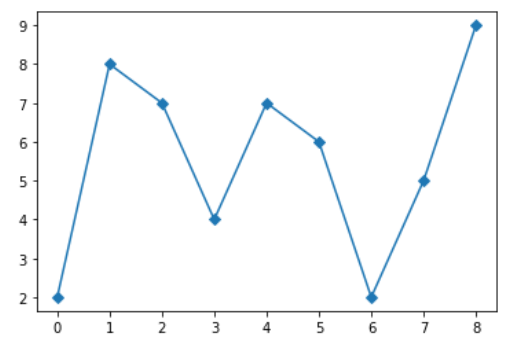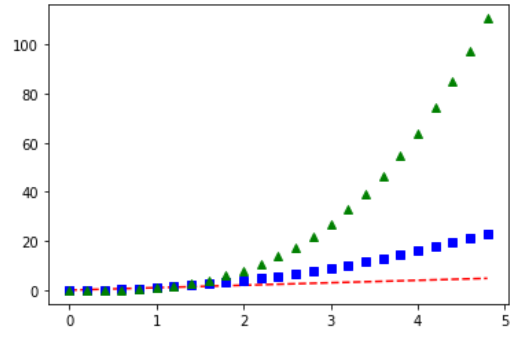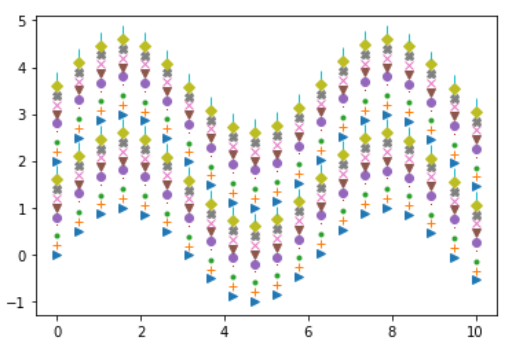Open in App
Not now

# How to Add Markers to a Graph Plot in Matplotlib with Python?

• Last Updated : 03 Oct, 2022

In this article, we will learn how to add markers to a Graph Plot in Matplotlib with Python. For that just see some concepts that we will use in our work.

• Graph Plot: A plot is a graphical technique for representing a data set, usually as a graph showing the relationship between two or more variables.
• Markers: The markers are shown in graphs with different shapes and colors to modify the meaning of the graph.

## Python3

 `# importing packages``import` `matplotlib.pyplot as plt` `# plot with marker``plt.plot([``2``, ``8``, ``7``, ``4``, ``7``, ``6``, ``2``, ``5``, ``9``], marker``=``'D'``)``plt.show()`

Output :## Python3

 `# importing packages``import` `matplotlib.pyplot as plt` `# create data``t ``=` `np.arange(``0.``, ``5.``, ``0.2``)` `# plot with marker``plt.plot(t, t, ``'r--'``, t, t``*``*``2``, ``'bs'``, t, t``*``*``3``, ``'g^'``)``plt.show()`

Output :## Python3

 `# importing packages``import` `matplotlib.pyplot as plt``import` `numpy as np` `# create data``x_values ``=` `np.linspace(``0``, ``10``, ``20``)``y_values ``=` `np.sin(x_values)``markers ``=` `[``'>'``, ``'+'``, ``'.'``, ``','``, ``'o'``, ``'v'``, ``'x'``, ``'X'``, ``'D'``, ``'|'``]` `# apply markers``for` `i ``in` `range``(``20``):``    ``plt.plot(x_values, y_values ``+` `i``*``0.2``, markers[i ``%` `10``])``plt.show()`

Output :My Personal Notes arrow_drop_up